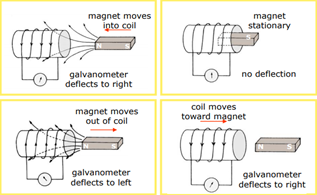# How Electromagnetic induction can be used to generate electricity### Introduction to Electromagnetic induction

Electromagnetic induction is the process of producing a voltage by using a magnetic field and current in a complete circuit. It was first discovered by Michael faraday he started his work by using a different combination of wires, magnetic strengths, and currents

Electromagnetic induction is created by moving a conductive substance through a magnetic field. When the magnet moves back and forth a current will be induced in the wire

### What is Magnetic Flux

It will be easy to understand electromagnetic induction if we know about the magnetic flux. The magnetic flux is depended upon the magnetic flux density and the coil, it is proportional to these two qualities. Magnetic flux is the product of magnetic flux density and the area normal to the lines of magnetic flux. Unit of magnetic flux is weber (Wb)

### How to calculate the amount of magnetic flux

The amount of magnetic flux can be calculated by the equation

• φ = BA cos θ
• φ = the amount of magnetic flux in webers (Wb)
• B = magnetic flux density
• A = cross-sectional surface area
• Θ = angle between the magnetic field lines and normal to the cross-sectional area

The flux we calculated is the flux through one turn of the coil in order to calculate the magnetic flux In all the turns of the coil, we need to use a quantity called magnetic flux linkage which can be defined as

• Nφ =NBA cos θ

N is the number of turnings

### How can we change the flux over a period of time

• To change the flux we can move the magnet or wire away or towards
• The area can be increased or decreased to change the flux
• A wire can be rotated along an axis which is perpendicular to the field thus changing the angle between the area and magnetic field vectors

### Factors affecting the magnitude of induced e.m.f

Larger current is produced

• If the magnet is moved at a faster speed in and out of the coil
• A Stronger magnet can be used
• Turns in the coil is increased
• The soft iron core can be inserted in the coil to increase the current

No E.M.F is produced

• If the magnetic field is stationary outside or inside the coil
• If there no relative movement between the magnet and the coil

### Electrical power generations

Electrical generators follow the same method to produce e.m.f by moving the conductor

### An electromagnetic blood flow meter

It is used to detect the flow rate of arterial blood, it uses the EMF induced in the flowing blood. A magnetic field is set up across the artery, and the connection is made to two small electrodes in contact with the artery

### Forever flashlight

It uses the EMF principle to eliminate the need for batteries

### Transformers

The primary coil current moves back and forth across the secondary coil the moving magnetic field caused by the changing field will create a current in the secondary coil

### Microphones

Sound waves enter the filter of a microphone, a diaphragm is situated in the filter the sound waves will vibrate the diaphragm which in turns moves the coil and the coil is wrapped around a magnet, so the movement of the wire in the magnet will create a current in the wire.